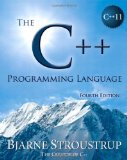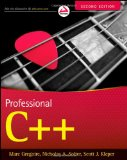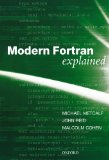Mixed language programming - C++11 and Fortran 2008

Posted on May 11, 2012 by Paul

The code for this tutorial is on GitHub: https://github.com/sol-prog/mix_fortran_cpp.

Different programming languages have different strengths and while you can express any imaginable algorithm in C++11 sometimes you need to interface your code with legacy codes written in Fortran, or you want to use modern Fortran for his rich matrix operations. This post will present some simple examples of mixed Fortran 2008 and C++11 code, as a side note it is entirely possible to write these examples in pure Fortran or C++, coding every operations from scratch in C++ or using matrix libraries like Eigen.

Mixing Fortran with C (or C++) was a painful experience in the past and the exact mechanism was compiler dependent. Starting with Fortran 2003 you can use a standardized mechanism for interoperability with C, calling Fortran from C and vice versa can be done using the iso_c_binding Fortran module.

Let’s start by writting a C++11 code that creates two N by N arrays filled with random numbers. In this post we use the column-major order storage (Fortran order) and not the typical row-major storage used in C or C++ for multidimensional arrays.

The iso_c_binding Fortran module defines numerical types compatible with C and C++ numerical types, e.g. a C int is defined as c_int and a C double as c_double. We are going to implement a Fortran subroutine, callable from C, that multiplies two matrices filled with doubles. For matrix multiplication we are going to use a Fortran intrinsic function named matmul:

Please note the use of bind(c) on line 1 and iso_c_binding module on line 2, the actual matrix multiplication is done on line 6.

In order to be able to use the above Fortran subroutine in C we need to translate Fortran’s subroutine definition to a C function:

became:

Please note that every variable is declared as a pointer, this is because Fortran uses call by reference by default.

What about a C++ definition for matrix_multiply ? We can use the C definition wrapped in an extern “C” declaration:

Now, we can modify our original C++ code in order to use matrix_multiply for multiplying A and B:

Please note the syntax used at line 50 for sending a C++ STL vector to our Fortran subroutine by reference.

The above codes can be compiled with gcc-4.7 on Linux and Mac or with Visual Studio and Intel Fortran on Windows.

If you save the Fortran code in fortran_matrix_multiply.f90 and C++ in cpp_main_1.cpp you can compile and link them on Unix:

On Windows open an Intel Fortran command prompt and write these two lines for compiling and linking the codes:

If you are interested in learning more about the new C++11 syntax I would recommend reading The C++ Programming Language by Bjarne Stroustrup.or, Professional C++ by M. Gregoire, N. A. Solter, S. J. Kleper 4th edition:If you need to brush your Fortran knowledge a good book is Modern Fortran Explained by M. Metcalf, J. Reid and M. Cohen: